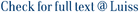This paper is concerned with the maximum cut problem in parallel on cubic graphs. New theoretical results characterizing the cardinality of the cut are presented. These results make it possible to design a simple combinatorial O(log n) time parallel algorithm, running on a CRCW P-RAM with O(n) processors. The approximation ratio achieved by the algorithm is 1·3 and improves the best known parallel approximation ratio, i.e. 2, in the special class of cubic graphs. The algorithm also guarantees that the size of the returned cut is at least ((9g −3)/8 g)n, where g is the odd girth of the input graph. Experimental results round off the paper, showing that the solutions obtained in practice are likely to be much better than the theoretical lower bound.

On max-cut in cubic graphs / Calamoneri, Tiziana; Finocchi, Irene; Manoussakis, J; Petreschi, Rossella. - In: PARALLEL ALGORITHMS AND APPLICATIONS. - ISSN 1063-7192. - 17:3(2002), pp. 165-183. [10.1080/10637190127724]

### On max-cut in cubic graphs

#### Abstract

This paper is concerned with the maximum cut problem in parallel on cubic graphs. New theoretical results characterizing the cardinality of the cut are presented. These results make it possible to design a simple combinatorial O(log n) time parallel algorithm, running on a CRCW P-RAM with O(n) processors. The approximation ratio achieved by the algorithm is 1·3 and improves the best known parallel approximation ratio, i.e. 2, in the special class of cubic graphs. The algorithm also guarantees that the size of the returned cut is at least ((9g −3)/8 g)n, where g is the odd girth of the input graph. Experimental results round off the paper, showing that the solutions obtained in practice are likely to be much better than the theoretical lower bound.
##### Scheda breve Scheda completa Scheda completa (DC)2002
Parallel algorithms, Approximation algorithms, Max cut, Bipartite graphs, Cubic graphs, P-RAM model
On max-cut in cubic graphs / Calamoneri, Tiziana; Finocchi, Irene; Manoussakis, J; Petreschi, Rossella. - In: PARALLEL ALGORITHMS AND APPLICATIONS. - ISSN 1063-7192. - 17:3(2002), pp. 165-183. [10.1080/10637190127724]
File in questo prodotto:
Non ci sono file associati a questo prodotto.
##### Pubblicazioni consigliate

I documenti in IRIS sono protetti da copyright e tutti i diritti sono riservati, salvo diversa indicazione.

Utilizza questo identificativo per citare o creare un link a questo documento: `https://hdl.handle.net/11385/192651`
##### Citazioni
•6
•6